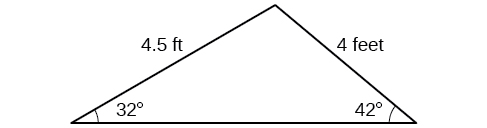## Section 6.1: Non-right Triangles: Law of Sines

### Learning Outcomes

By the end of this section, you will be able to:

• Use the Law of Sines to solve oblique triangles.
• Find the area of an oblique triangle using the sine function.
• Solve applied problems using the Law of Sines.

Suppose two radar stations located 20 miles apart each detect an aircraft between them. The angle of elevation measured by the first station is 35 degrees, whereas the angle of elevation measured by the second station is 15 degrees. How can we determine the altitude of the aircraft? We see in Figure 1 that the triangle formed by the aircraft and the two stations is not a right triangle, so we cannot use what we know about right triangles. In this section, we will find out how to solve problems involving non-right triangles.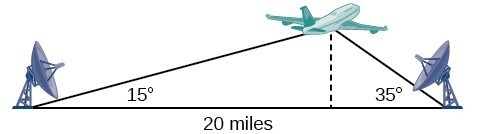Figure 1

## Using the Law of Sines to Solve Obliques Triangles

In any triangle, we can draw an altitude, a perpendicular line from one vertex to the opposite side, forming two right triangles. It would be preferable, however, to have methods that we can apply directly to non-right triangles without first having to create right triangles.

Any triangle that is not a right triangle is an oblique triangle. Solving an oblique triangle means finding the measurements of all three angles and all three sides. To do so, we need to start with at least three of these values, including at least one of the sides. We will investigate three possible oblique triangle problem situations:

1. ASA (angle-side-angle) We know the measurements of two angles and the included side. See Figure 2.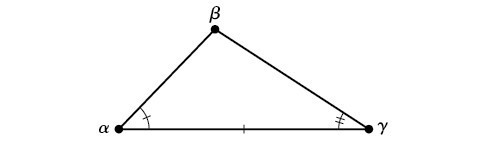Figure 2

2. AAS (angle-angle-side) We know the measurements of two angles and a side that is not between the known angles. See Figure 3.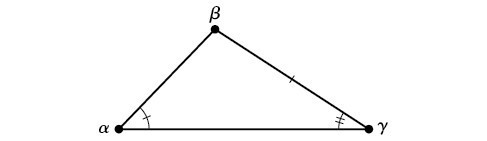Figure 3

3. SSA (side-side-angle) We know the measurements of two sides and an angle that is not between the known sides. See Figure 4.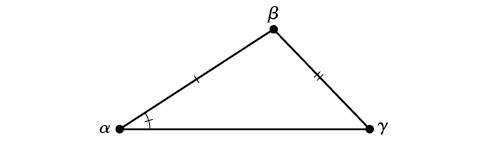Figure 4

Knowing how to approach each of these situations enables us to solve oblique triangles without having to drop a perpendicular to form two right triangles. Instead, we can use the fact that the ratio of the measurement of one of the angles to the length of its opposite side will be equal to the other two ratios of angle measure to opposite side. Let’s see how this statement is derived by considering the triangle shown in Figure 5.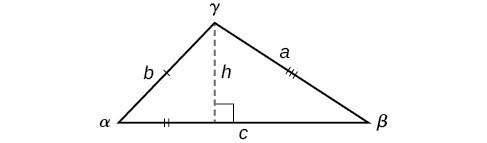Figure 5

Using the right triangle relationships, we know that $\sin \alpha =\frac{h}{b}$ and $\sin \beta =\frac{h}{a}$. Solving both equations for $h$ gives two different expressions for $h$.

$h=b\sin \alpha \text{ and }h=a\sin \beta$

We then set the expressions equal to each other.

\begin{align}b\sin \alpha &=a\sin \beta \\ \left(\frac{1}{ab}\right)\left(b\sin \alpha \right)&=\left(a\sin \beta \right)\left(\frac{1}{ab}\right) && \text{Multiply both sides by}\frac{1}{ab}. \\ \frac{\sin \alpha }{a}&=\frac{\sin \beta }{b} \end{align}

Similarly, we can compare the other ratios.

$\frac{\sin \alpha }{a}=\frac{\sin \gamma }{c}\text{ and }\frac{\sin \beta }{b}=\frac{\sin \gamma }{c}$

Collectively, these relationships are called the Law of Sines.

$\frac{\sin \alpha }{a}=\frac{\sin \beta }{b}=\frac{\sin \lambda }{c}$

Note the standard way of labeling triangles: angle $\alpha$ (alpha) is opposite side $a$; angle $\beta$ (beta) is opposite side $b$; and angle $\gamma$ (gamma) is opposite side $c$. See Figure 6.

While calculating angles and sides, be sure to carry the exact values through to the final answer. Generally, final answers are rounded to the nearest tenth, unless otherwise specified.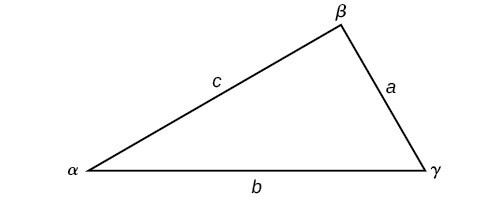Figure 6

### A General Note: Law of Sines

Given a triangle with angles and opposite sides labeled as in Figure 6, the ratio of the measurement of an angle to the length of its opposite side will be equal to the other two ratios of angle measure to opposite side. All proportions will be equal. The Law of Sines is based on proportions and is presented symbolically two ways.

$\frac{\sin \alpha }{a}=\frac{\sin \beta }{b}=\frac{\sin \gamma }{c}$

$\frac{a}{\sin \alpha }=\frac{b}{\sin \beta }=\frac{c}{\sin \gamma }$

To solve an oblique triangle, use any pair of applicable ratios.

### Example 1: Solving for Two Unknown Sides and Angle of an AAS Triangle

Solve the triangle shown in Figure 7 to the nearest tenth.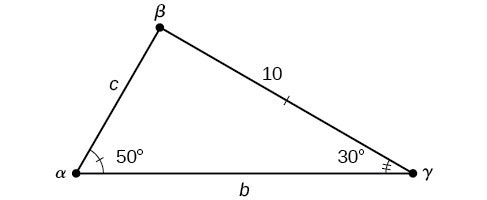Figure 7

### Try It

Solve the triangle shown in Figure 8 to the nearest tenth.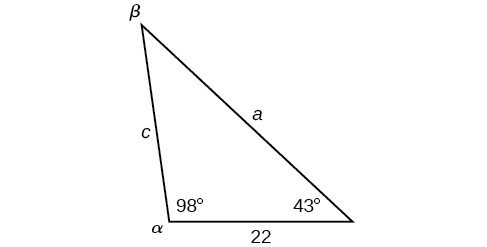Figure 8

## Using The Law of Sines to Solve SSA Triangles

We can use the Law of Sines to solve any oblique triangle, but some solutions may not be straightforward. In some cases, more than one triangle may satisfy the given criteria, which we describe as an ambiguous case. Triangles classified as SSA, those in which we know the lengths of two sides and the measurement of the angle opposite one of the given sides, may result in one or two solutions, or even no solution.

### A General Note: Possible Outcomes for SSA Triangles

Oblique triangles in the category SSA may have four different outcomes. Figure 9 illustrates the solutions with the known sides $a$ and $b$ and known angle $\alpha$.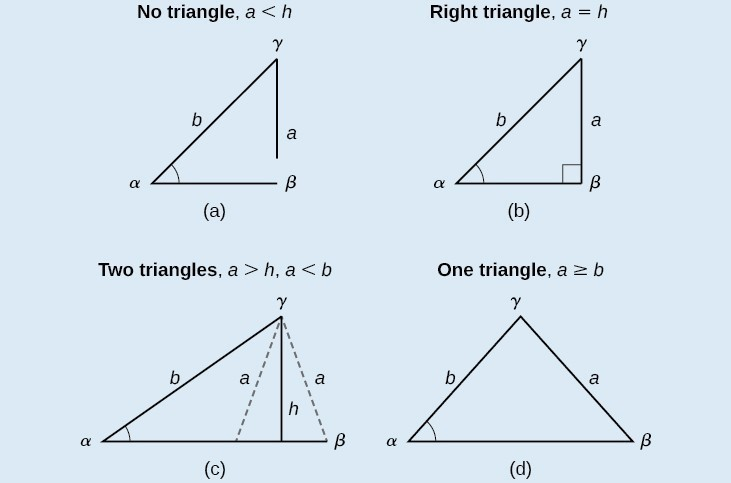Figure 9

### Example 2: Solving an Oblique SSA Triangle

Solve the triangle in Figure 10 for the missing side and find the missing angle measures to the nearest tenth.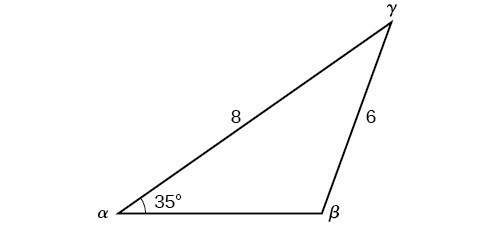Figure 10

### Try It

Given $\alpha =80^\circ ,a=120$, and $b=121$, find the missing side and angles. If there is more than one possible solution, show both.

### Example 3: Solving for the Unknown Sides and Angles of a SSA Triangle

In the triangle shown in Figure 13, solve for the unknown side and angles. Round your answers to the nearest tenth.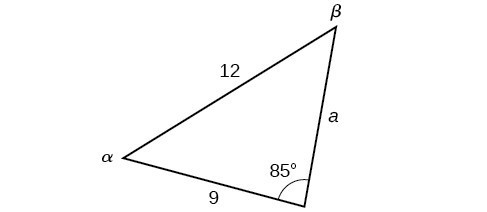Figure 13

### Try It

Given $\alpha =80^\circ ,a=100,b=10$, find the missing side and angles. If there is more than one possible solution, show both. Round your answers to the nearest tenth.

### Example 4: Finding the Triangles That Meet the Given Criteria

Find all possible triangles if one side has length 4 opposite an angle of 50°, and a second side has length 10.

### Try It

Determine the number of triangles possible given $a=31,b=26,\beta =48^\circ$.

## Finding the Area of an Oblique Triangle Using the Sine Function

Now that we can solve a triangle for missing values, we can use some of those values and the sine function to find the area of an oblique triangle. Recall that the area formula for a triangle is given as $\text{Area}=\frac{1}{2}bh$, where $b$ is base and $h$ is height. For oblique triangles, we must find $h$ before we can use the area formula. Observing the two triangles in Figure 15, one acute and one obtuse, we can drop a perpendicular to represent the height and then apply the trigonometric property $\sin \alpha =\frac{\text{opposite}}{\text{hypotenuse}}$ to write an equation for area in oblique triangles. In the acute triangle, we have $\sin \alpha =\frac{h}{c}$ or $c\sin \alpha =h$. However, in the obtuse triangle, we drop the perpendicular outside the triangle and extend the base $b$ to form a right triangle. The angle used in calculation is ${\alpha }^{\prime }$, or $180-\alpha$.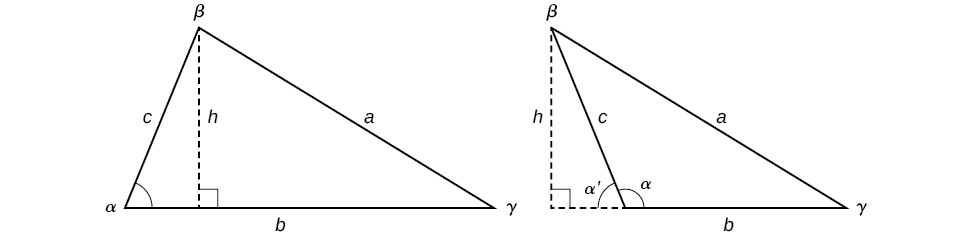Figure 15

Thus,

$\text{Area}=\frac{1}{2}\left(\text{base}\right)\left(\text{height}\right)=\frac{1}{2}b\left(c\sin \alpha \right)$

Similarly,

$\text{Area}=\frac{1}{2}a\left(b\sin \gamma \right)=\frac{1}{2}a\left(c\sin \beta \right)$

### A General Note: Area of an Oblique Triangle

The formula for the area of an oblique triangle is given by

\begin{align}\text{Area}&=\frac{1}{2}bc\sin \alpha \\ &=\frac{1}{2}ac\sin \beta \\ &=\frac{1}{2}ab\sin \gamma \end{align}

This is equivalent to one-half of the product of two sides and the sine of their included angle.

### Example 5: Finding the Area of an Oblique Triangle

Find the area of a triangle with sides $a=90,b=52$, and angle $\gamma =102^\circ$. Round the area to the nearest integer.

### Try It

Find the area of the triangle given $\beta =42^\circ ,a=7.2\text{ft},c=3.4\text{ft}$. Round the area to the nearest tenth.

## Solving Applied Problems Using the Law of Sines

The more we study trigonometric applications, the more we discover that the applications are countless. Some are flat, diagram-type situations, but many applications in calculus, engineering, and physics involve three dimensions and motion.

### Example 6: Finding an Altitude

Find the altitude of the aircraft in the problem introduced at the beginning of this section, shown in Figure 16. Round the altitude to the nearest tenth of a mile.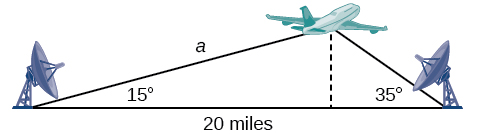Figure 16

### Try It

The diagram shown in Figure 17 represents the height of a blimp flying over a football stadium. Find the height of the blimp if the angle of elevation at the southern end zone, point A, is 70°, the angle of elevation from the northern end zone, point $B$, is 62°, and the distance between the viewing points of the two end zones is 145 yards.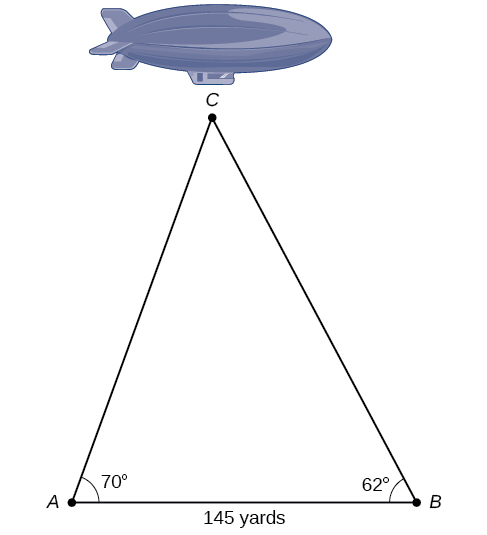Figure 17

## Key Equations

 Law of Sines $\frac{\sin \alpha }{a}=\frac{\sin \beta }{b}=\frac{\sin \gamma }{c}$ $\frac{a}{\sin \alpha }=\frac{b}{\sin \beta }=\frac{c}{\sin \gamma }$ Area for oblique triangles \begin{align}\text{Area}&=\frac{1}{2}bc\sin \alpha \\ &=\frac{1}{2}ac\sin \beta \\ &=\frac{1}{2}ab\sin \gamma \end{align}

## Key Concepts

• The Law of Sines can be used to solve oblique triangles, which are non-right triangles.
• According to the Law of Sines, the ratio of the measurement of one of the angles to the length of its opposite side equals the other two ratios of angle measure to opposite side.
• There are three possible cases: ASA, AAS, SSA. Depending on the information given, we can choose the appropriate equation to find the requested solution.
• The ambiguous case arises when an oblique triangle can have different outcomes.
• There are three possible cases that arise from SSA arrangement—a single solution, two possible solutions, and no solution.
• The Law of Sines can be used to solve triangles with given criteria.
• The general area formula for triangles translates to oblique triangles by first finding the appropriate height value.
• There are many trigonometric applications. They can often be solved by first drawing a diagram of the given information and then using the appropriate equation.

## Glossary

altitude
a perpendicular line from one vertex of a triangle to the opposite side, or in the case of an obtuse triangle, to the line containing the opposite side, forming two right triangles
ambiguous case
a scenario in which more than one triangle is a valid solution for a given oblique SSA triangle
Law of Sines
states that the ratio of the measurement of one angle of a triangle to the length of its opposite side is equal to the remaining two ratios of angle measure to opposite side; any pair of proportions may be used to solve for a missing angle or side
oblique triangle
any triangle that is not a right triangle

## Section 6.1 Homework Exercises

1. Describe the altitude of a triangle.

2. Compare right triangles and oblique triangles.

3. When can you use the Law of Sines to find a missing angle?

4. In the Law of Sines, what is the relationship between the angle in the numerator and the side in the denominator?

5. What type of triangle results in an ambiguous case?

For the following exercises, assume $\alpha$ is opposite side $a,\beta$ is opposite side $b$, and $\gamma$ is opposite side $c$. Solve each triangle, if possible. Round each answer to the nearest tenth.

6. $\alpha =43^\circ ,\gamma =69^\circ ,a=20$

7. $\alpha =35^\circ ,\gamma =73^\circ ,c=20$

8. $\alpha =60^\circ ,\beta =60^\circ ,\gamma =60^\circ$

9. $a=4,\alpha =60^\circ ,\beta =100^\circ$

10. $b=10,\beta =95^\circ ,\gamma =30^\circ$

For the following exercises, use the Law of Sines to solve for the missing side for each oblique triangle. Round each answer to the nearest hundredth. Assume that angle $A$ is opposite side $a$, angle $B$ is opposite side $b$, and angle $C$ is opposite side $c$.

11. Find side $b$ when $A=37^\circ ,B=49^\circ ,c=5$.

12. Find side $a$ when $A=132^\circ ,C=23^\circ ,b=10$.

13. Find side $c$ when $B=37^\circ ,C=21,b=23$.

For the following exercises, assume $\alpha$ is opposite side $a,\beta$ is opposite side $b$, and $\gamma$ is opposite side $c$. Determine whether there is no triangle, one triangle, or two triangles. Then solve each triangle, if possible. Round each answer to the nearest tenth.

14. $\alpha =119^\circ ,a=14,b=26$

15. $\gamma =113^\circ ,b=10,c=32$

16. $b=3.5,c=5.3,\gamma =80^\circ$

17. $a=12,c=17,\alpha =35^\circ$

18. $a=20.5,b=35.0,\beta =25^\circ$

19. $a=7,c=9,\alpha =43^\circ$

20. $a=7,b=3,\beta =24^\circ$

21. $b=13,c=5,\gamma =10^\circ$

22. $a=2.3,c=1.8,\gamma =28^\circ$

23. $\beta =119^\circ ,b=8.2,a=11.3$

For the following exercises, use the Law of Sines to solve, if possible, the missing side or angle for each triangle or triangles in the ambiguous case. Round each answer to the nearest tenth.

24. Find angle $A$ when $a=24,b=5,B=22^\circ$.

25. Find angle $A$ when $a=13,b=6,B=20^\circ$.

26. Find angle $B$ when $A=12^\circ ,a=2,b=9$.

For the following exercises, find the area of the triangle with the given measurements. Round each answer to the nearest tenth.

27. $a=5,c=6,\beta =35^\circ$

28. $b=11,c=8,\alpha =28^\circ$

29. $a=32,b=24,\gamma =75^\circ$

30. $a=7.2,b=4.5,\gamma =43^\circ$

For the following exercises, find the length of side $x$. Round to the nearest tenth.

31.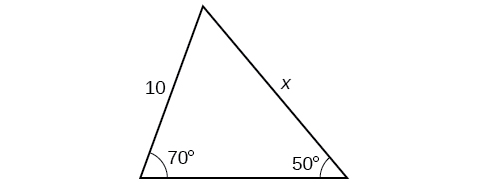32.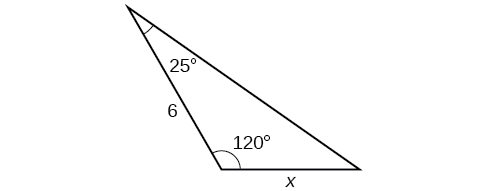33.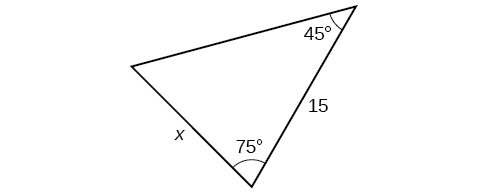34.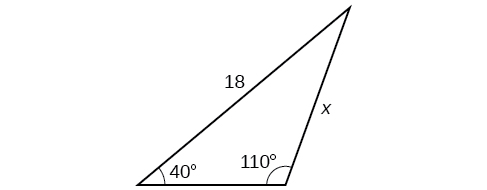35.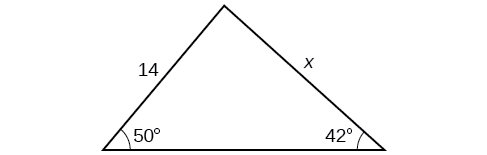36.For the following exercises, find the measure of angle $x$, if possible. Round to the nearest tenth.

37.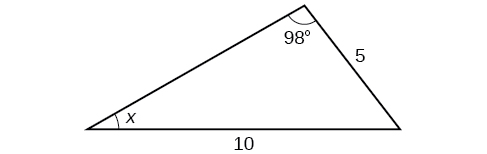38.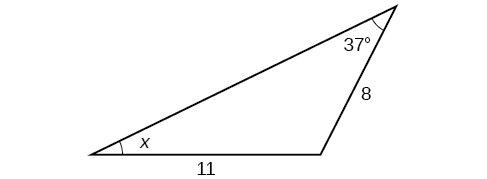39.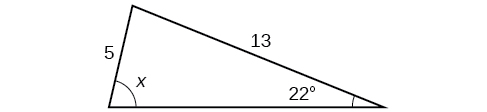40.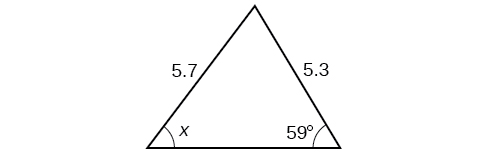41. Notice that $x$ is an obtuse angle.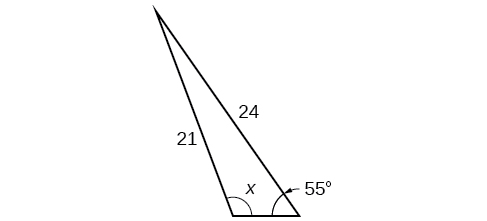42.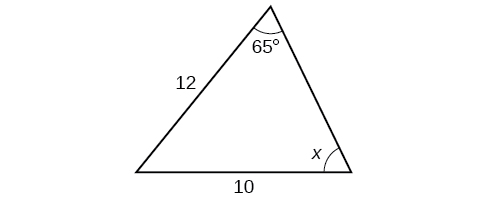For the following exercises, find the area of each triangle. Round each answer to the nearest tenth.

43.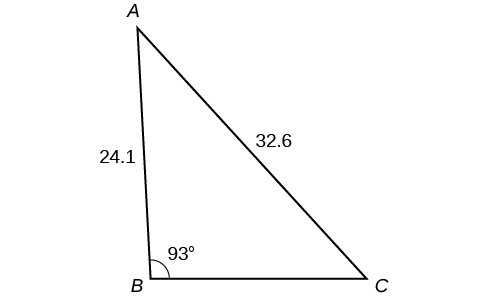44.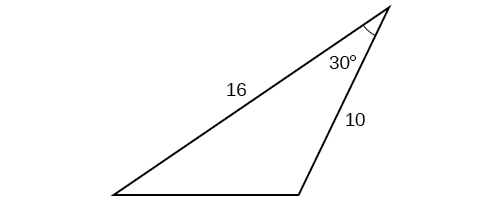45.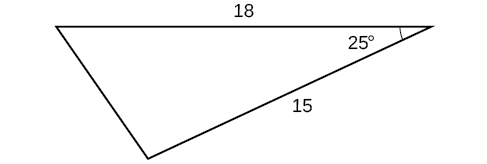46.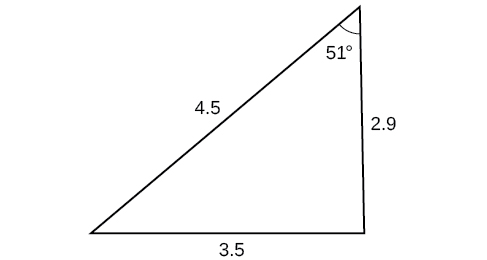47.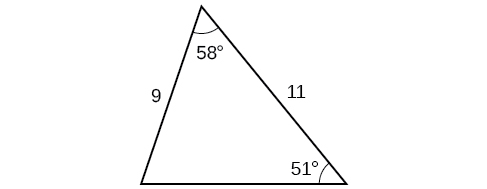48.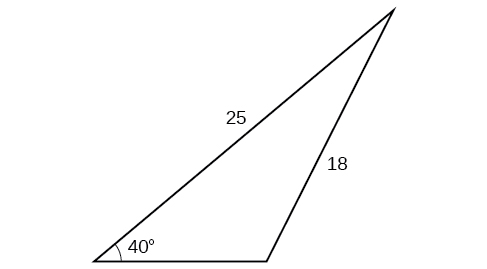49.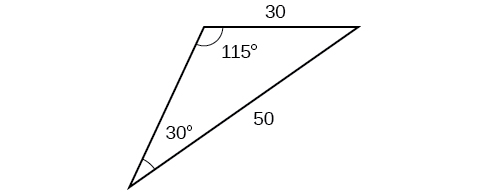50. Find the radius of the circle below. Round to the nearest tenth.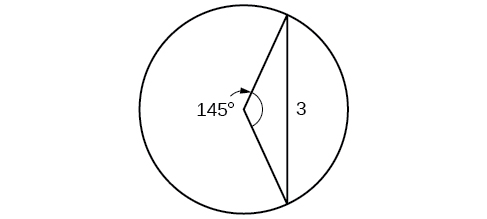51. Find the diameter of the circle below. Round to the nearest tenth.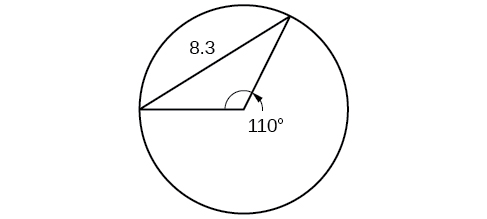52. Find $m\angle ADC$ in the figure below. Round to the nearest tenth.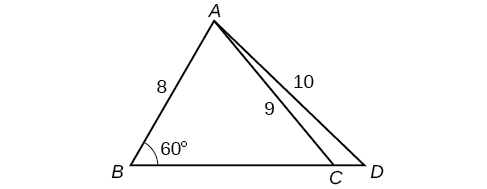53. Find $AD$ in the triangle below. Round to the nearest tenth.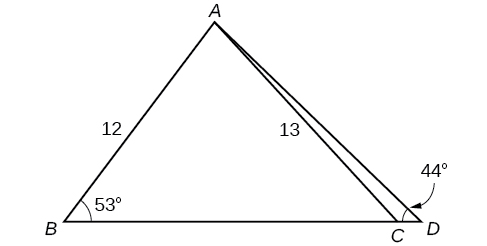54. Solve both triangles. Round each answer to the nearest tenth.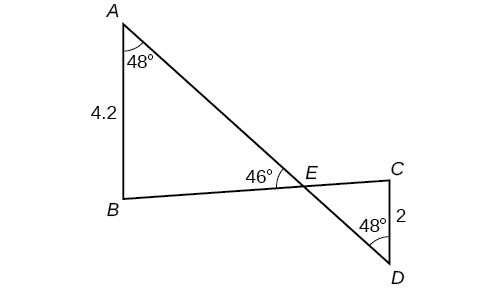55. Find $AB$ in the parallelogram below.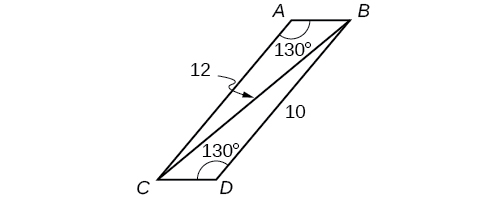56.  Solve the triangle below. (Hint: Draw a perpendicular from $H$ to $JK$). Round each answer to the nearest tenth.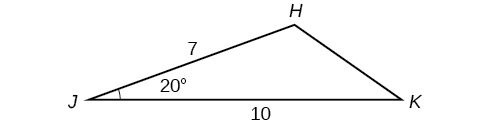57. Solve the triangle below. (Hint: Draw a perpendicular from $N$ to $LM$). Round each answer to the nearest tenth.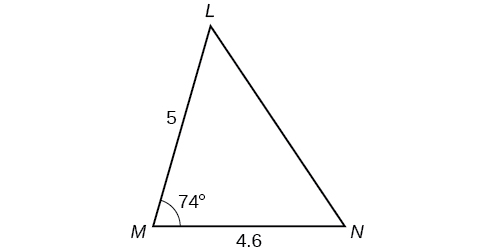58. In the figure below, $ABCD$ is not a parallelogram. $\angle m$ is obtuse. Solve both triangles. Round each answer to the nearest tenth.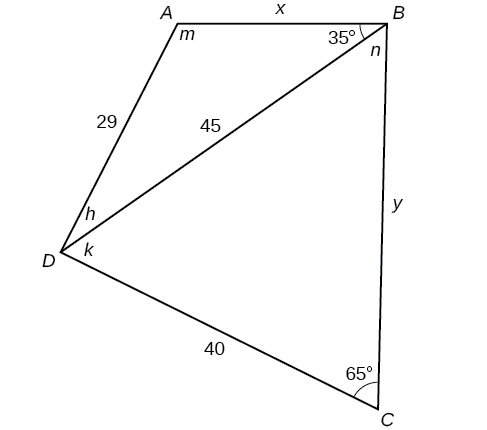59. A pole leans away from the sun at an angle of $7^\circ$ to the vertical, as shown in below. When the elevation of the sun is $55^\circ$, the pole casts a shadow 42 feet long on the level ground. How long is the pole? Round the answer to the nearest tenth.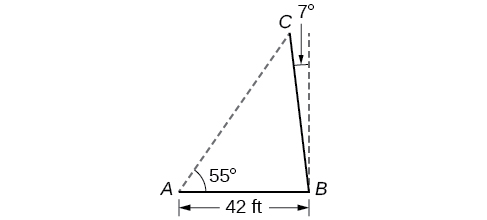60. To determine how far a boat is from shore, two radar stations 500 feet apart find the angles out to the boat, as shown below. Determine the distance of the boat from station $A$ and the distance of the boat from shore. Round your answers to the nearest whole foot.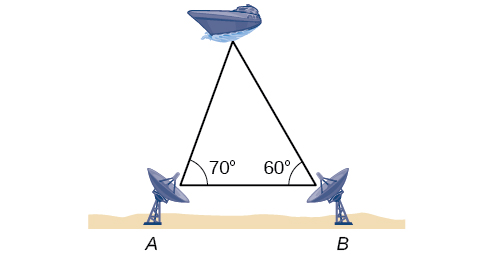61. The diagram below shows a satellite orbiting Earth. The satellite passes directly over two tracking stations $A$ and $B$, which are 69 miles apart. When the satellite is on one side of the two stations, the angles of elevation at $A$ and $B$ are measured to be $86.2^\circ$ and $83.9^\circ$, respectively. How far is the satellite from station $A$ and how high is the satellite above the ground? Round answers to the nearest whole mile.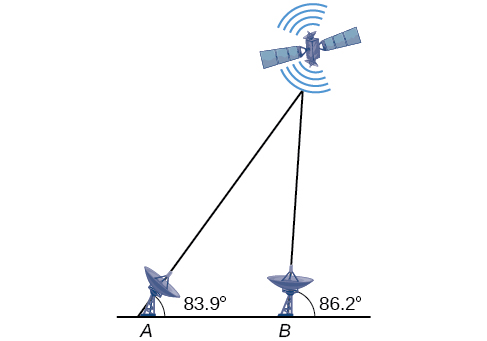62. A communications tower is located at the top of a steep hill, as shown below. The angle of inclination of the hill is $67^\circ$. A guy wire is to be attached to the top of the tower and to the ground, 165 meters downhill from the base of the tower. The angle formed by the guy wire and the hill is $16^\circ$. Find the length of the cable required for the guy wire to the nearest whole meter.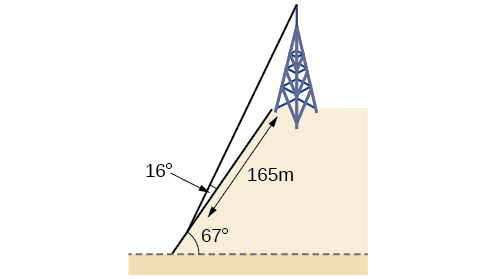63. The roof of a house is at a $20^\circ$ angle. An 8-foot solar panel is to be mounted on the roof and should be angled $38^\circ$ relative to the horizontal for optimal results. How long does the vertical support holding up the back of the panel need to be? Round to the nearest tenth.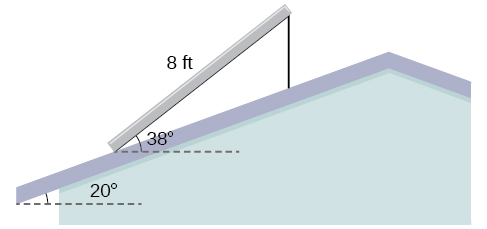64. Similar to an angle of elevation, an angle of depression is the acute angle formed by a horizontal line and an observer’s line of sight to an object below the horizontal. A pilot is flying over a straight highway. He determines the angles of depression to two mileposts, 6.6 km apart, to be $37^\circ$ and $44^\circ$, as shown below. Find the distance of the plane from point $A$ to the nearest tenth of a kilometer.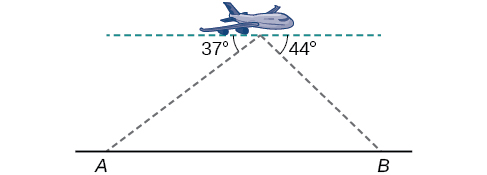65. A pilot is flying over a straight highway. He determines the angles of depression to two mileposts, 4.3 km apart, to be 32° and 56°, as shown below. Find the distance of the plane from point $A$ to the nearest tenth of a kilometer.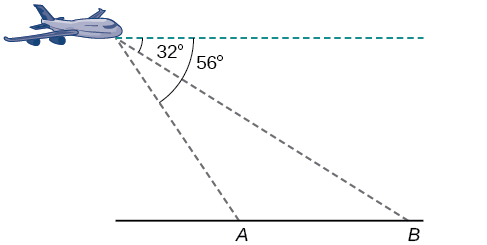66. In order to estimate the height of a building, two students stand at a certain distance from the building at street level. From this point, they find the angle of elevation from the street to the top of the building to be 39°. They then move 300 feet closer to the building and find the angle of elevation to be 50°. Assuming that the street is level, estimate the height of the building to the nearest foot.

67. In order to estimate the height of a building, two students stand at a certain distance from the building at street level. From this point, they find the angle of elevation from the street to the top of the building to be 35°. They then move 250 feet closer to the building and find the angle of elevation to be 53°. Assuming that the street is level, estimate the height of the building to the nearest foot.

68. Points $A$ and $B$ are on opposite sides of a lake. Point $C$ is 97 meters from $A$. The measure of angle $BAC$ is determined to be 101°, and the measure of angle $ACB$ is determined to be 53°. What is the distance from $A$ to $B$, rounded to the nearest whole meter?

69. A man and a woman standing $3\frac{1}{2}$ miles apart spot a hot air balloon at the same time. If the angle of elevation from the man to the balloon is 27°, and the angle of elevation from the woman to the balloon is 41°, find the altitude of the balloon to the nearest foot.

70. Two search teams spot a stranded climber on a mountain. The first search team is 0.5 miles from the second search team, and both teams are at an altitude of 1 mile. The angle of elevation from the first search team to the stranded climber is 15°. The angle of elevation from the second search team to the climber is 22°. What is the altitude of the climber? Round to the nearest tenth of a mile.

71. A street light is mounted on a pole. A 6-foot-tall man is standing on the street a short distance from the pole, casting a shadow. The angle of elevation from the tip of the man’s shadow to the top of his head of 28°. A 6-foot-tall woman is standing on the same street on the opposite side of the pole from the man. The angle of elevation from the tip of her shadow to the top of her head is 28°. If the man and woman are 20 feet apart, how far is the street light from the tip of the shadow of each person? Round the distance to the nearest tenth of a foot.

72. Three cities, $A,B$, and $C$, are located so that city $A$ is due east of city $B$. If city $C$ is located 35° west of north from city $B$ and is 100 miles from city $A$ and 70 miles from city $B$, how far is city $A$ from city $B?$ Round the distance to the nearest tenth of a mile.

73. Two streets meet at an 80° angle. At the corner, a park is being built in the shape of a triangle. Find the area of the park if, along one road, the park measures 180 feet, and along the other road, the park measures 215 feet.

74. Brian’s house is on a corner lot. Find the area of the front yard if the edges measure 40 and 56 feet.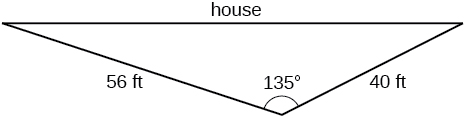75. The Bermuda triangle is a region of the Atlantic Ocean that connects Bermuda, Florida, and Puerto Rico. Find the area of the Bermuda triangle if the distance from Florida to Bermuda is 1030 miles, the distance from Puerto Rico to Bermuda is 980 miles, and the angle created by the two distances is 62°.

76. A yield sign measures 30 inches on all three sides. What is the area of the sign?

77. Naomi bought a modern dining table whose top is in the shape of a triangle. Find the area of the table top if two of the sides measure 4 feet and 4.5 feet, and the smaller angles measure 32° and 42°.